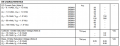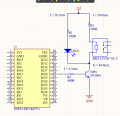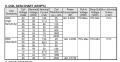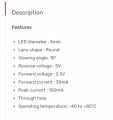How to read a transistor datasheet

Tiong

Joined Dec 5, 2019
3
https://www.onsemi.com/pdf/datasheet/2n3903-d.pdf

Link above are the datasheet for the transistor 2N3903/2N3094.

(1) May I ask if my Ic (Collector Current) is 35.7mA, what is the base current I should used?

(2) May I ask how do I read the gain from the table below ? Do I need to concern about this when calculating my Ib (base current)?

(3) For example my Ib (base current) is 4.3mA, what will the gain of the transistor be? Because there are multiple gain shown in the table.

Thanks in advance as I am stuck designing my circuit as i have no idea how to read the ON Characteristics table.Ian0

Joined Aug 7, 2020
4,146
from Figure 15 the gain at 35mA will be between 35% (at -55°C, 60% (at 25°C) and 80% (at 125°C) of the typical value at Vce = 1V.
The typical value (from the chart) is 150 for a 2N3903 and 300 for a 2N3904; and the minimum value is 50 (2N3903) or 100 (2N3904). The maximum value is not specified.

So at room temperature you can expect at least 30 @ 35mA for a 2N3903 and 60 for a 2N3904.
You should provide at least 35mA/30 = 1.16mA of base current.
If you want Vce to go lower than 1V, then you will need more base current.

At a base current of 4.3mA you can't determine the gain unless you know the collector current.

The morel of the story is that you can't rely on the gain being any particular value, at best you can determine the minimum value. So, don't design a circuit where actual value of Hfe is important for its operation.

•Tiong

MrSalts

Joined Apr 2, 2020
852
If you are using the transistor as a switch (all on, all off), a common practice is to assume a gain of 10 and use a base current that is 10% of the collector current.

If your transistor has high gain, and your switching speed (time from off to on state) isn't critical, you can use even lower base currents (5% or 2%). I would only worry about minimizing base current below 10% of collector current if you have a battery application or if your upstream circuitry is getting overloaded by driving too many "switch" transistors.
There are lower current options, if needed, like using MOSFET instead of BJT as switches but, until you clarify the application, I don't want to distract.

•Tiong and Hymie

BobTPH

Joined Jun 5, 2013
4,299
To add to what Ian has explained very well:

If you are using the transistor as a switch you use a bit more than the minimum base current to ensure that the transistor is fully on. A rule of thumb is to use the collector current/ 10 for low gain transistor and / 20 for higher gain ones.

I f you are using it in a linear application, you use negative feedback to control the actual gain well below what the data sheet says so that the desired gain can be achieved.

Bob

•Tiong

Ian0

Joined Aug 7, 2020
4,146
It is easier to understand the situation if you think of the transistor as a voltage controlled device that just happens to have an irritating diode connected between base and emitter that draws some current when things would be a lot easier if it didn't.
The collector current depends on the base voltage)
Just the same as a MOSFET is a voltage controlled device with an annoying capacitor. . . .

•Tiong

BobaMosfet

Joined Jul 1, 2009
1,917
https://www.onsemi.com/pdf/datasheet/2n3903-d.pdf

Link above are the datasheet for the transistor 2N3903/2N3094.
(1) May I ask if my Ic (Collector Current) is 35.7mA, what is the base current I should used?
(2) May I ask how do I read the gain from the table below ? Do I need to concern about this when calculating my Ib (base current)?
(3) For example my Ib (base current) is 4.3mA, what will the gain of the transistor be? Because there are multiple gain shown in the table.

Thanks in advance as I am stuck designing my circuit as i have no idea how to read the ON Characteristics table.

View attachment 252077
Along with what others have said, you need to be aware of the fact that manufactures create data sheets to make their component compare favorably against other brands of similar/same components. As such, you should know that manufacturers' TEST conditions are usually the ideal condition they recommend the component to be used at.

Title: Understanding Basic Electronics, 1st Ed.
Publisher: The American Radio Relay League
ISBN: 0-87259-398-3

Last edited:
•Tiong

Tiong

Joined Dec 5, 2019
3This is the design I was planning to do. Using ESP32 to control the ON/OFF of the Relay. Below is the datasheet of the relayOn the SRD standard, the relay I am using is 12V and the nominal current would be 37.5mA. Thus, I am using a resistor R3 to limit the current at 36.6mA ( Do I have to put the resistor here? ). Other than that, resistor R1 is used to limit the current for the LED as the datasheet says the forward voltage is 2.5V and forward current is 30mA. So the total current at the collector would be Ic = 64.9mA . From what I learn from the reply, the base current would be 6.49mA. Thus, resistor R2 is used to limit the current to 6.5mA. Assumption used in calculating the resistor value is : The Vce = 1V( Should I used the VCE(sat) value or the VCE = 1V? ) , and the VBE(sat) = 0.7V (as shown at the datasheet min=0.69V)
( https://einstronic.com/product/super-bright-led-5mm-white/ )Other than that, May I asked is there anything I need to changed on the design? And also when I am designing such circuit, what important detailed I need to look into in the transistor datasheet? sorry for such a long question as i am still a newbie in trying designing the circuit.

Lastly, thank You for everyone putting in effort teaching me the newbie, Really appreciate it!!

Alec_t

Joined Sep 17, 2013
12,477
is there anything I need to changed on the design?
Any relay coil has considerable inductance which generates a back-emf spike when the relay switches off. Unless the relay has a built-in suppressor you need to add a reverse-biased diode (e.g. a 1N4148) across the coil to suppress the spike and prevent damage to the transistor.

•Tiong

MrChips

Joined Oct 2, 2009
25,053
Your resistor values are not correct.

R1 = 300Ω is two low.
This is going to put about 40mA through the LED which is too high.
I would aim for 2-5mA.

R3 = 300Ω is too high.
This puts about 16mA through the relay which is not enough to energize it. You don't need R3.

•Tiong

Ian0

Joined Aug 7, 2020
4,146
A 12V relay is designed to have 12V across it, so if you are connecting it to 12V, you don't need a resistor.
If you connect 300Ω in series with the relay's own resistance of 320Ω, then you have 620Ω, and the current will be limited to 19.4mA.
But you DO need a diode to suppress the back EMF when the relay switches off. Use a diode with a voltage rating greater than your supply voltage (12V) and a current rating greater than the relay's operating current (37mA) so a 1N4148 rated at 75V/300mA will do fine.
With an LED forward voltage of 2.5V, there will be 10.5V across its resistor, so you would need 350Ω to limit it to 30mA (by the way, 30mA is a lot for an LED, it will probably be bright enough with 5mA)
6.49mA is correct for the base current. Use Vbe(SAT) because you want the transistor switched on as hard as it can be, that gives (3.3V-0.7V)/6.5mA = 400Ω. Correct again. Use 390Ω because it is a standard value.

If you were to taking absolutely everything into account, you would look up the output resistance of the ESP32's port (probably about 50Ω) and subtract that from your calculated value giving 350Ω, then use 330Ω as the nearest standard value.

•Tiong

crutschow

Joined Mar 14, 2008
28,557
It is easier to understand the situation if you think of the transistor as a voltage controlled device that just happens to have an irritating diode connected between base and emitter that draws some current when things would be a lot easier if it didn't.
The collector current depends on the base voltage)
Here we go again.
How is that easier?
Yes, the solid-state physics model shows that the BJT transistor acts as a voltage-controlled device, but the base-emitter voltage varies very little with a change in collector current, and also is temperature sensitive, so is of little use in calculating the DC bias conditions for a particular circuit.
For that purpose a black-box current-controlled model is usually used, which is why the current-gain values (beta or Hfe) are always specified in the data sheet.

The voltage-controlled (transconductance) model is mainly of use when the transistor is being using as a small-signal AC amplifier, which is not the case here.

•ericgibbs

Ian0

Joined Aug 7, 2020
4,146
How is that easier?
It's easier, because to use a transistor as a switch, one ignores all the Hfe graphs which vary all over the place with collector current and temperature and just looks at the lines on the datasheet which refer to saturation, and calculates a resistor value which is appropriate to give Vbe(sat) at the base at Ib(sat) into the base.

ericgibbs

Joined Jan 29, 2010
14,712
) May I ask if my Ic (Collector Current) is 35.7mA, what is the base current I should used?
Hi Tiong,
The data sheet for the 2N3904 Page #6 shows Ib versus Ic graphs, these are typical values

E

Attachments

• 85.5 KB Views: 3
•Tiong

crutschow

Joined Mar 14, 2008
28,557
It's easier, because to use a transistor as a switch, one ignores all the Hfe graphs which vary all over the place with collector current and temperature and just looks at the lines on the datasheet which refer to saturation, and calculates a resistor value which is appropriate to give Vbe(sat) at the base at Ib(sat) into the base.
No that's not how it's done.
You calculate a resistor value to give a base current of about 1/10th the maximum collector current.
Vbe(sat) is only used to subtract from the base source voltage to give that resistor value.
Getting an appropriate Vbe(sat) voltage never enters into that, as that naturally results from the base current.

Audioguru again

Joined Oct 21, 2019
4,129
The relay coil is 320 ohms and you added 300 ohms resistor in series producing a total resistance of 620 ohms.
12V/620 ohms= 19.4mA. The 320 ohms coil with a current of only 19.4mA has a voltage across it of only 320 ohms x 19.4mA= 6.2V. The coil needs at least 75% of 12V which is a minimum voltage of 9V so it will not work!

The absolute maximum current for the LED is 30mA when it is cool. If it is in a warm location then it will soon burn out!
Its brightness and forward voltage are rated at 20mA so use 20mA.
Its 10 or 15 degrees of viewing angle makes a beam that is too narrow to be an indicator, it is a spotlight almost like a laser beam.

Ian0

Joined Aug 7, 2020
4,146
No that's not how it's done.
You calculate a resistor value to give a base current of about 1/10th the maximum collector current.
Vbe(sat) is only used to subtract from the base source voltage to give that resistor value.
Getting an appropriate Vbe(sat) voltage never enters into that, as that naturally results from the base current.
So you mean that I don't take the supply voltage, subtract Vbe(sat) then divide by Ib?

crutschow

Joined Mar 14, 2008
28,557
So you mean that I don't take the supply voltage, subtract Vbe(sat) then divide by Ib?
Of course not. That's not what I meant or said (read my third sentence in post #14).
Don't know how you arrived at that odd conclusion(?).

My point is that the calculation has nothing to do with the BJT being a voltage operated device, which is the premise that started this side-bar.
You are calculating a base current, using the current operated model.
The Vbe(sat) is only used to determine the desired resistor value to get the desired base current.

Audioguru again

Joined Oct 21, 2019
4,129
I doubt the 3.3V powered processor can produce exactly 3.3V when loaded by the transistor's base current. The calculation for the series base resistor must allow for the voltage drop.

Ian0

Joined Aug 7, 2020
4,146
I doubt the 3.3V powered processor can produce exactly 3.3V when loaded by the transistor's base current. The calculation for the series base resistor must allow for the voltage drop.
I guessed at an output resistance of about 50Ω back in post #10.

Ian0

Joined Aug 7, 2020
4,146
You are calculating a base current, using the current operated model.
Or I could be calculating the base current based on the amount of current that would flow through the B-E diode at Vbe(sat), and that the base current depends only on the base-emitter diode, and the collector current depends on the base-emitter voltage.
If this exchange proves anything, it is that either method would result in the same answer in this case.
That being the case I believe it is more useful to think of a bipolar transistor as a voltage controlled device because MOSFETs, and JFETs are also voltage controlled devices.